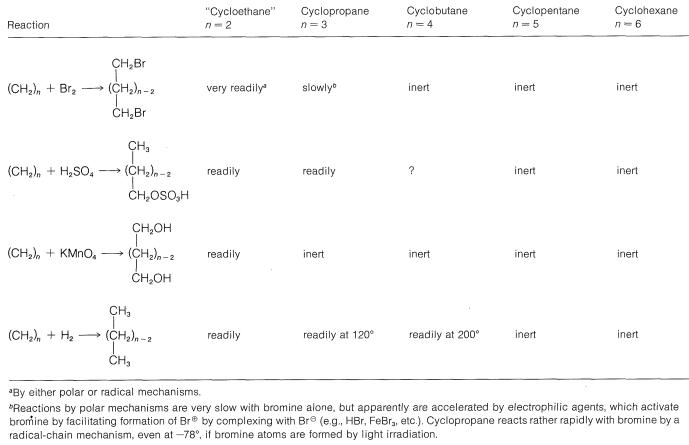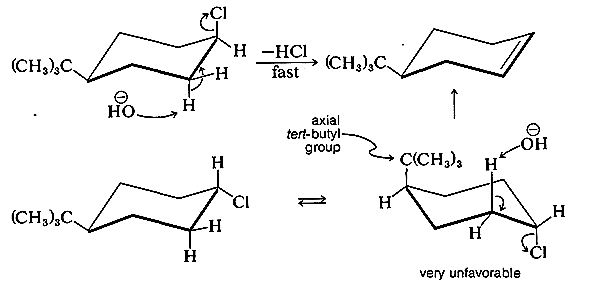# 12.5: Chemical Properties

$$\newcommand{\vecs}{\overset { \rightharpoonup} {\mathbf{#1}} }$$ $$\newcommand{\vecd}{\overset{-\!-\!\rightharpoonup}{\vphantom{a}\smash {#1}}}$$$$\newcommand{\id}{\mathrm{id}}$$ $$\newcommand{\Span}{\mathrm{span}}$$ $$\newcommand{\kernel}{\mathrm{null}\,}$$ $$\newcommand{\range}{\mathrm{range}\,}$$ $$\newcommand{\RealPart}{\mathrm{Re}}$$ $$\newcommand{\ImaginaryPart}{\mathrm{Im}}$$ $$\newcommand{\Argument}{\mathrm{Arg}}$$ $$\newcommand{\norm}{\| #1 \|}$$ $$\newcommand{\inner}{\langle #1, #2 \rangle}$$ $$\newcommand{\Span}{\mathrm{span}}$$ $$\newcommand{\id}{\mathrm{id}}$$ $$\newcommand{\Span}{\mathrm{span}}$$ $$\newcommand{\kernel}{\mathrm{null}\,}$$ $$\newcommand{\range}{\mathrm{range}\,}$$ $$\newcommand{\RealPart}{\mathrm{Re}}$$ $$\newcommand{\ImaginaryPart}{\mathrm{Im}}$$ $$\newcommand{\Argument}{\mathrm{Arg}}$$ $$\newcommand{\norm}{\| #1 \|}$$ $$\newcommand{\inner}{\langle #1, #2 \rangle}$$ $$\newcommand{\Span}{\mathrm{span}}$$$$\newcommand{\AA}{\unicode[.8,0]{x212B}}$$

Strain in small-ring cycloalkanes has a profound influence on their heats of combustion (Table 12-3). We reasonably expect that other chemical properties also will be affected. Indeed, like alkenes, cyclopropane and cyclobutane undergo $$\ce{C-C}$$ bond cleavage reactions that are not observed for cyclopentane and cyclohexane, or for saturated, open-chain hydrocarbons. A summary of these reactions is presented in Table 12-4. It will be seen that the reactions result in cleavage of a $$\ce{C-C}$$ bond to give an open-chain compound with normal bond angles. Relief of angle strain is an important contributing factor to the driving force for these reactions. Therefore, ethene is highly reactive, whereas cyclopropane and cyclobutane are somewhat less reactive. The $$\ce{C-C}$$ bonds of the larger, relatively strain-free cycloalkanes are inert, so these substances resemble the alkanes in their chemical behavior. Substitution reactions, such as chlorination of cyclopentane and higher cycloalkanes, generally are less complex than those of the corresponding alkanes because there are fewer possible isomeric substitution products. Thus cyclohexane gives only one monochloro product, whereas hexane gives three isomeric monochloro hexanes.

Conformation has a major influence on the chemical reactivity of cycloalkanes. To understand its effect in any one reaction, we first need to know what the conformation is of the transition state, and this requires a knowledge of the reaction mechanism. Next, we have to decide what amount of energy is required for the reactants to achieve transition-state conformations. For example, consider the $$E_2$$ elimination discussed in Section 8-8D. The preferred transition state requires the leaving groups to be antarafacial and coplanar:For cyclohexane derivatives to react in this way, the transition-state conformation must have both leaving groups axial:Table 12-4: Ring-Cleavage Reactions of CycloalkanesFor this reason, compounds such as cis-4-tert-butylchlorocyclohexane eliminate $$\ce{HCl}$$ much more readily by the $$E_2$$ mechanism than do the corresponding trans isomers.To have the antarafacial coplanar mechanism of cycloalkanes operate with the trans isomer, the transition state would have to have the tert-butyl group in the highly unfavorable axial position.